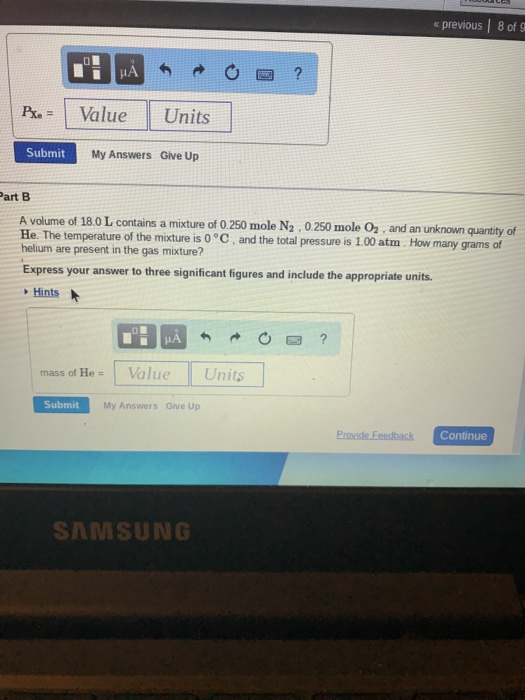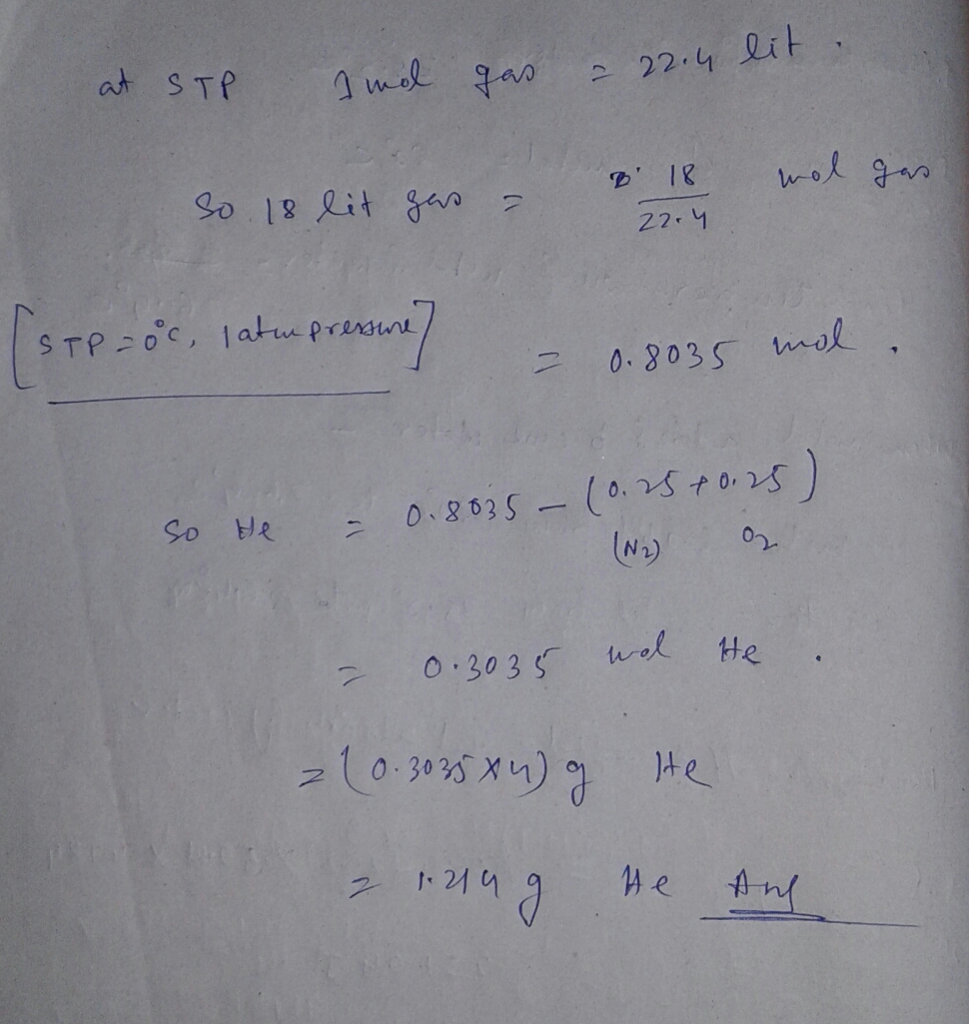# Question & Answer: A volume of 18.0 L contains a mixture of 0.250 mole N_2, 0.250 mole O_2, and an unknown…..A volume of 18.0 L contains a mixture of 0.250 mole N_2, 0.250 mole O_2, and an unknown quantity of He. The temperature of the mixture is 0 degree C, and the total pressure is 1.00 atm. How many grams of helium are present in the gas mixture? Express your answer to three significant figures and include the appropriate units. Mass of He =